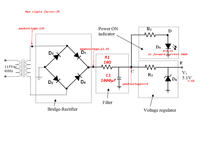# Power Supply Circuit.

Status
Not open for further replies.

#### Ethan221009

##### Newbie level 3
How to choose the right component inorder to get a 5.1V +-5% output voltage?do not worry about the selection of the components used in your circuit!
you can use typical components to develop your circuit and you do not need special components.

good luck

•Zhi Xian

### Zhi Xian

Points: 2
I'm guessing this is a school question. It isn't difficult to deduce the components from the figures given:

R1 has 1.3V dropped across it and it's value is 10 Ohms. Using Ohms law the current through it can be worked out (I = V/R) = 1.3/10 = 0.13A or 130mA.

40mA is lot of current for a power indicator LED but the only figure given so assume R2 must drop 11V down to 4.1V at 40mA so it's value is (R = V/I) = 6.9/0.04 = 172.5 Ohms

Assuming no other current is drawn from the output, the current NOT flowing through R2 must be 130 - 40mA = 90mA so if D6 is a 5.1V Zener diode, R2 drops 11V - 5.1V at 90mA making it (R = V/I) = 5.9/.09 = 65.55 Ohms.

Brian.

I'm guessing this is a school question. It isn't difficult to deduce the components from the figures given:

R1 has 1.3V dropped across it and it's value is 10 Ohms. Using Ohms law the current through it can be worked out (I = V/R) = 1.3/10 = 0.13A or 130mA.

40mA is lot of current for a power indicator LED but the only figure given so assume R2 must drop 11V down to 4.1V at 40mA so it's value is (R = V/I) = 6.9/0.04 = 172.5 Ohms

Assuming no other current is drawn from the output, the current NOT flowing through R2 must be 130 - 40mA = 90mA so if D6 is a 5.1V Zener diode, R2 drops 11V - 5.1V at 90mA making it (R = V/I) = 5.9/.09 = 65.55 Ohms.

Brian.

Thank you hamidrezakarami and betwixt for the advises and clear explanation. I kind of work out some of the calculation and it is almost the same but only the Output part with max load current of 80mA.

How to choose the right diode for D1, D2, D2 D4 with VF 0.7V? (My selection is 1N4001 With VRRM = 50V Io = 1A VF = 0.7V)

LED VF 4.1V at forward current of 40m VBR of -10V. (The Selection for R2 E96 13.7 1%tolerance = Max13.837 x 10)
To bring down the Maximum load current of the output to 80mA, can i use R2 to get this 130mA - 40mA = 80mA. (Will 50mA affect my LED light which operates at VF 40mA?)

Zener Diode at 5.1V 5 +-5% and Maximum Load current for Output is 80mA. (The Selection for R3 E96 73.2ohm 1%tolerance = Max 73.932ohm x 1) ( 1N4733 for Zener Diode selection)1N4001 is a good choice. The part is not critical and most siicon rectifiers will have a Vf of around 0.7V. In this kind of application, the cost is more important than exact ratings and 1N400x series are mass produced so their cost is very low. As long as the voltage rating is at least 2.8 times the peak AC voltage from the transformer so 50V PIV is OK. The current through the diodes is the output current of the circuit plus the surge current to initially charge the capacitor so again 1A rating is sufficient. You shuld note that the 1A rating is continuous current, for short periods it can be much higher so it will easily cope with the capacitor charge which will only take a fraction of a second.

It is unusual for a power indcator LED to need that much current or to have a Vf of 4.1V, a more typical LED would be around 1.6V at 10mA but lets stay with the figures provided. The LED current will be constant when fed from 11V so the resistor has to be 172.5 Ohms to give exactly that current. The nearest standard resistor value is 180 Ohms which would give a slightly lower current of 38.3mA which is still within tolerance.

The Zener diode is there to ensure the current through through R3 stays constant under different load conditions so it has to carry any of the 80mA that is NOT flowing out to the load. If you imagine a situation where 80mA was being drawn, the value of R3 could be set so it dropped exactly 11 - 5.1V and gave 5.1V at thre output without the Zener diode being present at all. However, if the load current was reduced, the voltage dropped in R3 would be less and the voltage would rise. The Zener is there to sink the extra current so 80mA always flows through R3 and therefore the output voltage stays constant. Looking at the worst case where no load is connected and the Zener has to sink all the current by itself, it would dissipate (W= VxI) = 5.1 x 0.08 = 0.408 Watts of power. The 1N4733 is rated at 1W so it would be a suitable type to use.

Brian.

Hi Brian,

D1-D4 - 1N4001
R2 - (E12)180ohm 10% tolerance
R3 - (E12)68 Ohms 10% tolerance
D6 - 1N4733

Ethan

Those values are fine - good luck!

Brian.

Status
Not open for further replies.